###Author Topic: What is the Ballistics Coefficient?  (Read 3686 times)

#### rsterne##### What is the Ballistics Coefficient?
« on: November 25, 2016, 11:21:46 AM »
I keep seeing a lot of misunderstanding of what the Ballistics Coefficient is.... In simple terms, it is how the drag of your projectile compares to that of a "Standard Projectile", such as the G1.... There are many standard projectiles, some of which are shown below....

Standard G1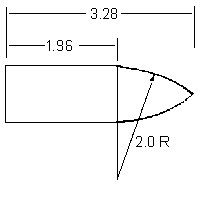Boattail (G7)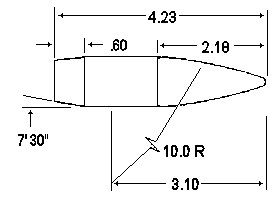Sphere (GS)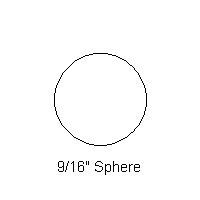Cylinder (GC)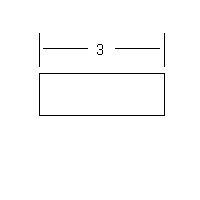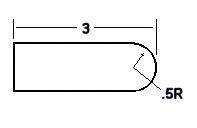.22LR (RA4)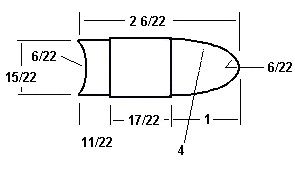These widely differing projectiles have completely different drag profiles.... The drag profile is simply a graph of what the Drag Coefficient is at various velocities.... Here are a few, at velocities below 2000 fps....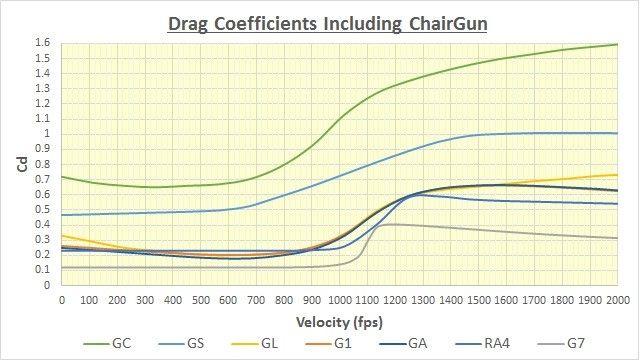Note that in every case, there is a huge increase in drag at in the Transonic region, which is Mach 0.8 to Mach 1.2.... The GA (Airgun) profile is a modification of the standard (widely accepted) G1 profile, and seems to be something peculiar to the ChairGun program.... In the next post, I will explain what the BC is and how it is calculated from the Cd....

• Coalmont, BC

#### rsterne##### Re: What is the Ballistics Coefficient?
« Reply #1 on: November 25, 2016, 11:58:49 AM »
OK, so now you understand that different shapes have different drag at any given velocity.... and a higher Cd indicates more drag.... You also understand that how the drag of an object changes, as you change the velocity, is a function of its shape.... In addition, it is clear that bad things happen to the drag between Mach 0.8 and 1.2 for every object.... This is one of the primary considerations for airguns.... because we have such limited power available it makes little sense to drive something Supersonic only to have it fall Subsonic again within a short distance and waste all that hard earned energy.... but that is a topic for another discussion....

We can measure how a projectile slows down in a couple of ways.... We can use two Chronographs some known distance apart, or we can use a Doppler Radar to measure directly the decay in velocity.... We can also use the trajectory, and if accurately done, along with an accurate muzzle velocity, we can calculate the velocity loss using the drop, but that adds another step and another source of error.... Whatever method you use, at some point in the calculations, whatever program or calculator you are using figures out the Drag Coefficient (Cd) between the two velocities.... What happens after that depends on which drag model you decide to compare that Cd to.... Every standard projectile has a different drag, at a given velocity, remember.... so which one we compare it to makes a big difference.... Here is a graph showing some standard drag profiles between 300 and 1500 fps, and I have added the bullet we need a BC for.... We determined that it has a Cd of 0.3 at 900 fps, and it is represented by the black dot....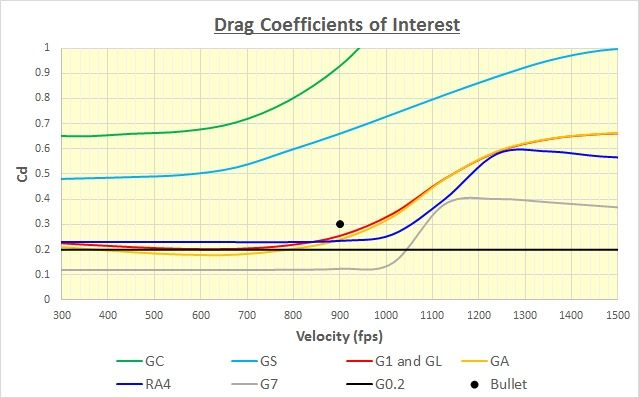A couple of things to note here.... In this velocity range, the G1 and GL Drag Models are virtually identical, so I just used the red line for both.... You can see how similar the GA Model (orange line) is to both of them.... In addition, I added the "G0.2" Drag Model (which assumed a constant Cd of 0.2), which years ago (in the first version of ChairGun, for example) was used for airgun pellets.... You can see that while it is pretty good below 800 fps, it totally ignores the rapid rise in drag in the Transonic and Supersonic regions, which is why it has been dropped by most.... OK, so we know the Cd of our projectile, how do we convert that to the BC ?....

The BC is composed of two parts, the Sectional Density and the Form Factor.... The SD is a measure of how heavy the bullet is compared to its caliber.... A high SD bullet has more mass pushing a given frontal area through the air, so for a given amount of drag, it will slow down less.... The FF is a measure of how "slippery" the bullet is compared to the standard projectile we are comparing it to.... For a moment, let's look at the G1 projectile....In addition to the proportions shown above, the standard G1 projectile is 1.000" caliber and weighs 1.000 lbs.... It therefore has an SD of 1.000.... since SD = Wt. (lbs) / Cal (in) ^2.... and by definition the FF is 1.000 (it's the standard, remember).... Let's say you exactly scale it down to half size.... Now it's .500 cal and (using the same density) it would weigh 0.125 lbs (0.500^3 = 0.125).... The SD would now be (0.125 / 0.500^2) = 0.125 / 0.250 = 0.500.... so the SD is also half what the standard was.... Note that if you keep the shape and density the same, that SD is proportional to the caliber.... However, the FF is unchanged (it's still the same shape).... so the FF still = 1.000.... Now let's look at the BC of our standard G1 projectile....

The BC = SD / FF .... so for our standard G1 projectile.... BC = 1.000 / 1.000 = 1.000.... Makes sense, right, after all, it IS the standard, so now we know how the BC is defined.... For our half size version.... BC = 0.500 / 1.000 = 0.500.... Note that just as the SD is proportional to caliber, so is the BC, if we don't change the shape or density of the projectile.... These are important concepts to grasp, so I'll let you chew on those while I write the next post....

Bob
« Last Edit: November 26, 2016, 09:52:17 AM by rsterne »
• Coalmont, BC

#### rsterne##### Re: What is the Ballistics Coefficient?
« Reply #2 on: November 25, 2016, 01:14:00 PM »
OK, so now we have a way to calculate the BC, if we know the Cd and the SD of a given projectile.... All we need to do is compare the Cd of our bullet with that of a standard bullet THAT HAS THE SAME SD, and at the same velocity.... They have to have the same SD or you would be comparing apples to oranges.... Remember;

BC = SD / FF .... so if you have an SD of 0.10, if the FF is the same as your standard projectile, the BC would be = 0.10 / 1.00 = 0.10.... Let's pick a bullet with an SD of 0.10.... that works out to 34 gr. in a .22 cal.... or it might be 44 gr. in .25 cal.... or 63 gr. in .30 cal.... or 86 gr. in .35 cal.... or 142 gr. in .45 cal.... It doesn't matter, as long as the SD is 0.10.... If the FF is the same as our standard projectile, then at a given velocity the Cd of our test bullet is the same as the Cd of the standard.... Effectively, this means that for any given velocity, the FF of our test bullet is Cd(test) / Cd(standard).... If our test bullet has less drag at that velocity, its FF will be less than one, so its BC will be greater than the standard bullet, providing they have the same SD and velocity.... If our test bullet has more drag than the standard, the FF will be greater than one, so the BC will be poorer than the standard bullet.... When FF = 1.000, then BC = SD, a very handy thing to remember....

We measured the velocity decay, and at an average velocity of 900 fps we determined that the Cd of our test bullet was 0.30.... I'll repost the chart here for convenience.... The black dot is our test bullet....OK, so a quick glance at that graph shows that our test bullet has less drag than a cylinder or sphere (GC or GS).... but more drag than any of the other standard projectiles.... If they were all the same SD, then relative to each different standard, our test bullet would have a different FF.... and therefore a different BC.... depending on which standard we compared it to.... In fact that is exactly the case.... In the table below are the BCs of our test bullet, calculated for each of the different standards.... Remember, all these widely varied BCs are for the SAME bullet, just compared to a different standard.... In every case the SD = 0.10....

Model         BC
GC           0.310
GS           0.220
G1           0.085
GL           0.085
GA           0.080
RA4         0.078
G0.2        0.067
G7           0.041

Now you can see why knowing the BC means nothing if you don't know what standard was used to calculate it.... It gets even worse.... Let's say you are shooting a roundball, so you should be using the GS standard.... If you used a BC of 0.220, and the GS standard, in ChairGun, you would get an accurate prediction of the drag of your roundball at other velocities.... so you would know how it performed as it slows down going downrange.... However, let's say you used the G7 drag model, and a BC of 0.041.... Although the data would be correct at 900 fps, as the velocity decayed as your roundball went downrange, the calculations would be off, because the G7 profile says the Cd is constant below 900 fps, but in fact if you look at the GS profile you will see that the drag drops about 25% as the bullet slows down.... If you used a BC of 0.041, with the G7 profile, for your roundball, by the time it had slowed to 600 fps you would be using too low a BC, so the trajectory would actually be flatter than what you calculated....

The purpose of this thread was not to make you fearful of using BCs because they are useless, not at all.... It was to hopefully help you understand why it is important to choose a drag profile that closely matches your bullet, if you wish to get accurate data at widely differing velocities than where you measured the BC.... However, if you are interested in what is happening at a specific distance, and you measure your BC at that distance, you are good to go.... It is VERY important, however to know what Drag Model was used to calculate the BC.... If you calculate it using, say, the G1 profile, and then try and look at the trajectory using the GS profile, you will get very wrong answers.... The BC you calculate will vary widely depending on the Drag Model you pick.... In the above example, the BC is anywhere between 0.041 and 0.310.... but remember, that in this example, at 900 fps with SD = 0.10.... BC(GC) of 0.310 = BC(G7) of 0.041....

I hope I didn't confuse you totally........ I will now unlock this thread to answer any questions you have, but please stay on topic....

Bob
« Last Edit: November 25, 2016, 01:23:03 PM by rsterne »
• Coalmont, BC

#### Christopher

• Sr. Member
•• Posts: 402##### Re: What is the Ballistics Coefficient?
« Reply #3 on: November 26, 2016, 04:06:25 AM »
The question that I have for you is about form factor.

I understand that shape has major bearing on how well it cuts through the atmosphere, due to the aerodynamics of the projectile.
Quote
The FF is a measure of how "slippery" the bullet is compared to the standard projectile we are comparing it to

But here is where I get lost is here:
Quote
In addition to the proportions shown above, the standard G1 projectile is 1.000" caliber and weighs 1.000 lbs.... It therefore has an SD of 1.000.... since SD = Wt. (lbs) / Cal (in) ^2.... and by definition the FF is 1.000 (it's the standard, remember)

I'm probably missing something obvious, but how did you derive at that number for the form factor? And not just for your example, but how do you determine form factor on any projectile? Take like the .250 cal 51 gr. BBT (SD of .116) you designed, would you just have to shoot it and plot trajectory to determine FF, or are there knowns about that bullet that help determine FF before shooting it?

Thanks,
Chris

#### rsterne##### Re: What is the Ballistics Coefficient?
« Reply #4 on: November 26, 2016, 09:14:07 AM »
The Form Factor of a standard projectile is, by definition, 1.000.... The Ballistics Coefficient, Sectional Density, and Form Factor are related by the following equation....

BC = SD / FF  .... and the SD is the weight in pounds divided by the caliber in inches squared....  SD = [Wt.(gr) / 7000] / [Cal. (in) x Cal. (in)]

Therefore, for the standard G1 projectile, which weighs 1 lb. and has a caliber of 1 in....

SD = 1.000 / (1.000 x 1.000) = 1.000 .... and therefore the BC = 1.000 / 1.000 = 1.000

I suppose you could pick a different Form Factor, but the purpose of having a standard is to make things easy and understandable, so why not have all the numbers end up being 1.000, right?....  We don't really care what the Form Factor is, it's a convenient way to show that the BC comes from two components, the SD and the "shape" (FF)....

Since the Drag Coefficient (Cd) is what is important, and since that changes with velocity.... unless your bullet is virtually identical to the standard you are using the FF is a fleeting thing anyway, just like the BC.... Pick any two curves on the graph, and compare their Cd at different velocities.... Let's go back to that roundball, which will follow the light blue line on the graph.... If we look at 900 fps, the Cd of the roundball is about 0.66, but for the G7 (boattail, the grey lline) it is only 0.12.... To make things easy, let's say the roundball has an SD of 0.10, so that BC can be found by using....

FF = Cd(test) / Cd(standard) .... Since BC = SD / FF then BC = 0.10 / (Cd(test) / Cd(standard) which simplifies to BC = Cd(standard) / Cd(test) / 10

If we are using the GS standard (sphere), with Cd = 0.66 (at 900 fps), and we are indeed using a roundball (wth the same Cd), then we have.... BC = 0.66 / 0.66 / 10 = 0.10

However if we want the BC using the G7 (boattail) as the standard, which has a Cd of just 0.12 at 900 fps.... then at that velocity we have.... BC = 0.12 / 0.66 / 10 = 0.018

In other words, at 900 fps, our roundball has a BC(GS) of 0.10.... but a BC(G7) of only 0.018.... Both statements are equally correct.... Now let's see what happens when we change the velocity, however.... Let's use 400 fps.... The Cd of the GS standard is now only 0.48, but the Cd of the G7 standard is still 0.12.... Since we are using a roundball, at 400 fps it will have a Cd of 0.48.... So using the same calculations, but at 400 fps, we have....

BC(GS) = 0.48 / 0.48 / 10 = 0.10 .... but BC(G7) = 0.12 / 0.48 / 10 = 0.025

If we do the same thing at 1200 fps, now the Cd(GS) = 0.86 and the Cd(G7) = 0.40.... Our roundball will have a Cd of 0.86, so at 1200 fps, we have....

BC(GS) = 0.86 / 0.86 / 10 = 0.10 .... but BC(G7) = 0.40 / 0.86 / 10 = 0.047

This shows what happens when you use the wrong standard projectile for your BC calculations.... While they are correct at any given velocity, the BC changes as you change the velocity.... but if you use the correct standard projectile (one that matches your bullet shape), the BC is constant.... For our roundball....

Velocity    BC (GS)   BC (G7)

400            0.10         0.025
900            0.10         0.018
1200          0.10         0.047

There are some very complex equations that break the shape of a bullet up into its components, and then calculate the drag of each surface, and add them up to figure out the total drag.... The best known is Robert McCoy's "McDrag" program, which is used by Geoffrey Kolbe and JBM Ballistics in their Drag Calculators.... I converted it into an Excel spreadsheet for my own use, and it is invaluable help in designing my Bob's Boattails.... It doesn't give the Form Factor (because that will be different, depending on what standard you compare to), it spits out the actual Cd, which is what is important anyway.... Once you know the Cd, you can calculate the BC at a given velocity.... but if your bullet isn't a very good match to the standard, your BC will vary with velocity anyway.... The very best way is to use a Doppler Radar to measure the velocity decay with distance, calculate from that the actual Cd at each velocity, and then plot that to make your own "Standard Drag Profile" for your own bullet.... That is what I hope to do, eventually....

Bob

« Last Edit: November 26, 2016, 09:55:49 AM by rsterne »
• Coalmont, BC

#### Christopher

• Sr. Member
•• Posts: 402##### Re: What is the Ballistics Coefficient?
« Reply #5 on: November 27, 2016, 02:31:39 AM »
Thanks Bob, like I said, I was missing something obvious. I appreciate you taking the time to say it in "slow motion" for me.

Chris